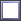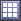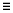# Review Evaluation Results

Review the input variable and output response values for each run, as well as review the run files.

## View Cross-Validation R2 History Summary

View a detailed cross-validation R2 history summary of all output responses in a tabular format using the R2 History tool.

1. From the Evaluate step, click the R2 Plot tab.
2. From the Channel selector, select the channels to display in the table.
3. Analyze the iteration history summary.

## Analyze R2 Plot

Plot the iteration history of cross-validation R2 value for each output response in a 2D chart using the R2 Plot tab.

1. From the Evaluate step, click the R2 Plot tab.
2. From the Channel selector, select the output response to plot R2 along the y-axis.
The x-axis respresents the iteration history.
3. Analyze the plot.

## View Run Data Summary

View a detailed summary of all input variable and output response run data in a tabular format from the Summary post processingEvaluation Data tab.

1. From the Evaluate step, click the Evaluation Data tab.
2. From the Post Processing step, click the Summary tab.
3. From the Channel selector, select the channels to display in the summary table.
4. Analyze the run data summary.
5. Optional: Disable run data from post processing without deleting it entirely from the study by clearing a run's corresponding checkbox in the Post Process column.
When a run is disabled, it will be removed from all plots, tables, and calculations in the Post Processing step.

## Analyze Evaluation Plot

Plot a 2D chart of the input variable and output response values for each run using the Evaluation Plot tool.

1. From the Evaluate step, click the Evaluation Plot tab.
2. From the Channel selector, select the input variable and/or output response to plot along the y-axis.
The x-axis represents the run numbers.
3. Analyze the plot.

## Analyze Dependency Between Two Sets of Data

Analyze the dependency between two sets of data in a scatter plot from the Scatter post processingEvaluation Scatter tab. Visually emphasize data in the scatter plot by appending additional dimensions in the form of bubbles.

1. From the Evaluate Step, click the Evaluation Scatter tab.
2. From the Post Processing step, click the Scatter tab.
3. Select data to display in the scatter plot.
• Use the Channel selector to select two dimensions of data to plot.
• Use the Correlation selector to select one or more values from the correlation map to plot.

Correlation measures the strength and direction between associated variables. Correlation coefficients can have a value from -1 to 1; -1 indicates a strong but negative correlation and 1 indicates a strong and positive correlation.

Note: Data points are colored according to their corresponding cell in the correlation map when there are no selections active in the Bubbles selector.
• Use the Bubbles selector to select additional dimensions of data to visually emphasize in the scatter plot. The selected input variables/output responses are represented by varying sizes and colors of bubbles.
The size and color of bubbles is determined by values in the run data for the selected input variable/output response. For size, larger bubbles equal larger values. For color, different shades of red, blue, and gray are used to visualize the range of values. The darker the shade of red, the larger the value. The lighter the shade of blue, the smaller the value. Gray represents the median value.
4. Analyze the dependencies between the selected data sets.
Tip: Display selected data in a single plot or separate plots by switching the Multiplot option between(single plot) and(multiple plots).
Configure the scatter plot's display settings by clicking(located in the top, right corner of the work area). For more information about these settings, refer to Evaluation Scatter Tab Settings.

### Evaluation ScatterScatter Tab Settings

Settings to configure the plots displayed in the Evaluation ScatterScatter post processing tab.

In the Scatter post processing tab, there are three methods for selecting data to display in the scatter plot: Channel, Correlation, and Bubble.

In the Evaluation Scatter tab, there are two methods for selecting data to display in the scatter plot: Channel and Bubble.

Access settings from the menu that displays when you click(located in the top, right corner of the work area).

#### Channel Settings

X-Bounds
Display the X bounds in the plot.
Y-Bounds
Display the Y bounds in the plot.

#### Correlation Settings

Pearson Product-Moment / Spearman's Rank
Pearson Product-Moment (default)
Assumes a linear association, and the coefficient values indicate how far away all of the data points are from a line of best fit through the data.
Spearman's Rank
Assumes a monotonic association, and the coefficient values indicate the degree of similarity between rankings.
Pearson and Spearman's correlation coefficients are shown in the following data set:
 -12 1 10 800 40 1200 1000 2000
 Figure 7. Pearson's Product-Moment Correlation Coefficient. Correlation coefficient is 0.82. There is a correlation but it is not perfectly linear. Figure 8. Spearman's Rank Correlation Coefficient. Correlation coefficient is 1.0. It is perfectly monotonic
Correlation ≥
Show only the column/rows with cells over the specified threshold.
Show Variables and Responses
Restrict the view of the entire correlation matrix to input variables only, output responses only, input variables and output responses, or input variables versus output responses.
X-Bounds
Display the X bounds in the plot.
Y-Bounds
Display the Y bounds in the plot.

#### Bubble Settings

Size
Scale
Adjust the overall size of all bubbles.
Focus
Adjust the size of bubbles so that smaller bubbles become smaller, while larger bubbles remain fixed, enabling the view to be directed at larger bubbles.
Invert
Reverse the size of bubbles so that smaller values are represented by larger bubbles.
Color
Discrete Steps
Change the level of color shading applied to bubbles.
Bins
Specify the number of red, blue, and gray shades used to color bubbles.
Invert
Reverse the color of bubbles so that red represents smaller values and blue represents larger values.